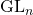#### Categories

1. algebraic geometry
2. algebraic topology
3. analysis of PDEs
4. category theory
5. classical analysis and ODEs
6. combinatorics
7. commutative algebra
8. complex variables
9. differential geometry
10. dynamical systems
11. functional analysis
12. general mathematics
13. general topology
14. geometric topology
15. group theory
16. information theory
17. K-theory and homology
18. logic
19. mathematical physics
20. metric geometry
21. number theory
22. numerical analysis
23. operator algebras
24. optimization and control
25. probability
26. quantum algebra
27. representation theory
28. rings and algebras
29. spectral theory
30. statistics theory
31. symplectic geometry

## Simple Proof of Tokuyama’s Formula

Tokuyama’s Formula is a combinatorial result that expresses the product of the deformed Weyl denominator and the Schur polynomial as a sum over strict Gelfand-Tsetlin patterns. This result implies Gelfand’s parametrization of the Schur polynomial, Weyl’s Character Formula, and Stanley’s formula on Hall-Littlewood polynomials — all for; also, the formula is related to alternating sign matrices. …